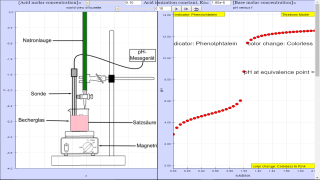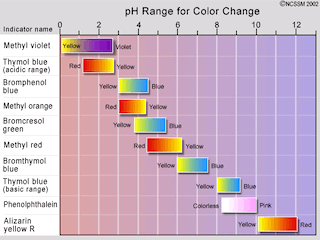Titrations

### Translations

Code Language Translator Run### Software Requirements

SoftwareRequirements

 Android iOS Windows MacOS with best with Chrome Chrome Chrome Chrome support full-screen? Yes. Chrome/Opera No. Firefox/ Samsung Internet Not yet Yes Yes cannot work on some mobile browser that don't understand JavaScript such as..... cannot work on Internet Explorer 9 and below

### CreditsFernando Fernandes; lookang

[text]

### For Teachers

The Titrations JavaScript Model is a JavaScript program that demonstrates the importance of computer simulation as a complement of experimental work. A typical students training in a chemistry laboratory is the titration of an acidic solution by a padronized base in order to determine the acid concentration in the sample. If the titration is followed by a visual method, a suitable indicator has to be previously chosen to detect the end point of the titration (the so-called equivalence point) by the sudden change of the indicator color. This is a common practice (alternative to using a pH meter)and does not imply the errors that may be thought on the subjective aspect of a color change, providing that the right indicator is chosen. This application is restricted to monoprotic acids and monofunctional strong bases.

Take a flask with a known volume (VA) of an aqueous solution of a monoprotic acid whose concentration is unknown. In a buret there is a padronized (well-known molar concentration) aqueous solution of a monofunctional strong base (sodium hydroxide, for example). Add to the flask a few drops of a suitable visual indicator and start pouring, slowly, the base over the acid.

The titration end point (equivalence point) is detected by a sudden change of the indicator color. Then, the buret scale indicates the volume of the base (VB) used in the neutralization reaction, and as its molar concentration, [Base], is well-known the corresponding number of moles is NB=VB*[Base]/1000. This number is equal to the number of acid moles, NA=NB, that have reacted and as the sample volume is VA, the acid molar concentration is worked out: [Acid] = NA*1000/VA. Therefore, the titration coordinate (f=NB/NA) is equal to 1 at the equivalence point.

The indicator is chosen accordingly to its effectiveness color range, that is, the equivalence point pH should be approximately at the middle of that range.

The simulations determine the pH at the equivalence point and choose the proper indicator from a table included in the program. The acid is characterized by the ionization constant (Ka); the default value is for acetic acid. As the base is assumed strong (fully ionized) its constant (Kb) is not a program input. The pH at equivalence point depends on Ka, [Base] and also on [Acid]. But the acid concentration is precisely what we want to know! Of course, the indicator choice requires a previous trial and error study to screen possible concentration bands of the acidic sample and assess whether or not the same indicator is appropriate for each band. To this end, different simulations should be done by changing the [Acid] and [Base] fields for a given acid. The program can probe wide ranges of Ka, from 10- 10 (very weak acids like hydrocyanic acid and phenol) to 109 (very strong acids like hydrochloric and sulphuric acids), and of [Acid] and [Base].

### Credits

The Titrations JS Model was developed by Fernando M.S. Silva Fernandes (Centre for Structural Chemistry, Faculty of Sciences, University of Lisboa) using version 5.1 of the Easy Java Simulations (EJS) modeling tool which can create stand alone JavaScript programs that run in almost any PC browser, tablets and smartphones. Information about EJS is available at: http://www.um.es/fem/Ejs/, and in the OSP ComPADRE collection: http://www.compadre.org/osp/.

### Model

Let's take the volume VA (in cm3) of an aqueous solution of a monoprotic acid HA (acetic acid, for example) of molar concentration CA. Then, the number of moles (NA) of the acid in VA is: NA=VA*CA/1000.

Let's drop over the acid a certain number of moles (NB), of a strong monofunctional base (sodium hydroxide, for example), following the procedure by the titration coordinate, f(i)=NB/NA.

Let's change f(i) from 0 to 2 with increments of 0.05, resulting in a titration with 41 steps ( i =0, 1, 2,..., 40). Therefore, step 20 corresponds to the equivalence point.

From NB and the molar concentration of the base (CB), the total volume of the base (VB) at the end of each step is: VB=1000*NB/CB, so the total volume of the mixture (VT) is: VT=VA+VB. It follows that the total concentrations of acid and base in the mixture at the end of each titration step, supposing that have not yet reacted, are, respectively: AT=1000*NA/VT and BT=1000*NB/VT.

As the titration has 41 steps, we should work out a titration curve with 41 pH values, stored in the array pH(i), in function of f(i).

Before the equivalence point (0⋜ f(i) <1) the titration process obeys the equation:

[H3O+]3 + (Ka+BT) * [H3O+]2 + (KA*BT - Ka*AT - Kw)*[H3O+] - Ka*Kw = 0 (BEP)

where [H3O+] is the hydronium ion concentration, Ka the acid ionization constant and Kw the water ionic product. AT and BT were defined above. Of course, the only unknown is [H3O+].

Resolving this equation with the different values of AT and BT, the values of pH=- log [H3O+] are determined for the titration process before the equivalence point.

At equivalence point and afterwards (f(i) ⋝ 1):

a) when f(i)=1, NA moles of salt are formed; this is the equivalence point.

b) when f(i)>1, the NA moles of salt, and the NB-NA moles of base in excess have to be taken into account.

The concentrations of salt, and of the base in excess, are respectively: ST=1000*NA/VT and BE=1000*(NB-NA)/VT. The salt and the strong base are totally ionized. The salt, however, undergoes hydrolysis, except in the titrations of strong acids by strong bases; this is why the pH, at the equivalence point, is generally different from 7. The titration process for f(i) ⋝ 1 obeys the equation:

[H3O+]3 + (BE+ST+Ka) * [H3O+]2 - (KW-BE *Ka)*[H3O+] - Ka*Kw = 0 (EPAEP)

Resolving this equation with the values of ST and BE, the pH's are determined for the titration process at, and after the equivalence point.

### A few computational detais

The equations are numerically solved by the method of successive bisections.

Once the pH at the equivalence point is determined, the appropriate indicator is chosen from a table included in the code. Both are displayed at the bottom of the plotting panel. The respective color change of the indicator is also displayed at the panel top.

The default variable values are for acetic acid (Ka=1.85E-5), with CA=0.10M, VA=50 cm3 and CB=0.10M.

The respective fields, however, can be edited. Try, for example, Ka=1E9 for a strong acid or/and change the values of the other variables. To this end, please Reset the simulation and actualize each new value by pressing the Return/Enter key.

The program has been tested in a wide range of Ka's: from 10- 10 (for very weak acids like hydrocyanic acid and phenol) to 109 (for very strong acids like hydrochloric and sulphuric acids). Nonetheless, if any inconsistency is found, please let us know.

- Incidentally, has the value of VA any influence on the titration results? Why?

- As the model is intended for strong bases, sodium hydroxide (NaOH) was chosen. Changing the name to another strong base, KOH for example, would it alter the results? Why?

- In textbooks, at least, the pH of a given solution is generally assumed as being between o and 14. Can it not be less than 0, or greater than 14? Why?

Finally, a suggestion: the code can be generalized to comprise weak bases and polifunctional acids and bases.

### Derivation of model equations

Before the equivalence point (0⋜ f(i) <1), we have to consider the following chemical equilibria (eq):

HA + H2O ⇌ A- + H3O+ for which the acid ionization constant is defined:

Ka=[A-]eq [H3O+]eq/[HA]eq (1)

and the water auto-ionization

H2O + H2O ⇌ H3O+ + OH- for which the ionic product constant is defined:

Kw=[H3O+]eq [OH-]eq (2)

As the base (NaOH, for example) is strong, it is totally ionized and the OH-ions from it react with the H3O+ions from the acid. The species electrically charged are A- , H3O+,OH- and Na+. The total acid concentration in the mixture (AT, see Introduction) is:

AT = [HA]eq + [A-]eq (3)

Additionally, the number of positive charges must be equal to the number of negative ones (electroneutrality condition), so:

BT + [H3O+]eq =[A-]eq + [OH-]eq (4)

where BT is the total base concentration in the mixture (see Introduction).

From equations (1), (2), (3) and (4), by simple algebraic manipulation, it is easy to eliminate all [...]eq, excepting [H3O+]eq, leading to equation (BEP) of the Introduction:

[H3O+]3 + (Ka+BT) * [H3O+]2 + (KA*BT - Ka*AT - Kw)*[H3O+] - Ka*Kw = 0 (BEP)

As for the process at, and after the equivalence point (f(i) ⋝ 1), the salt (NaA), totally ionized, generally undergoes hydrolysis and the base in execess (NaOH) is also assumed completely ionized. Then, the chemical equilibria to be considered are:

A- + H2O ⇌ HA + OH-

for which the hydrolysis constant is defined:

Kh=[HA]eq [OH-]eq/[A-]eq = Kw/Ka (5)

and, again, the water auto-ionization and respective ionic product constant.

Now, the chemical balances are:

ST=[A-]eq + [HA]eq (6)

and

BE+ST+[H3O+]eq = [OH-]eq + [A-]eq (7)

being the last the electroneutrality condition. BE and ST (see Introduction) are, respectively, the concentration of the salt at the equivalence point and the concentration of the base in excess.

Subtracting equations (6) and (7):

[HA]eq = [OH-]eq - [H3O+]eq - BE (8)

Now, eliminating all [...]eq but [H3O+]eq from equations (2), (5), (7) and (8), leads to equation (EPAEP) of the Introduction:

[H3O+]3 + (BE+ST+Ka) * [H3O+]2 - (KW-BE *Ka)*[H3O+] - Ka*Kw = 0 (EPAEP)

### Method of successive bisections

In order to work out the roots of the continuous algebraic equations of the model by the bisection method, it is necessary to make an initial guess of the intervals of the hydrogen ion concentration, (X1,X2), where the roots can be found. In the present particular case, such a guess is made from the very physical conditions of the problem. Indeed, the only interesting roots are real and positive and are between well-defined limits.

The minimum of the interval, X1, is always X1=0 for there are no negative concentrations. As for he maximum of the interval, X2, before the equivalence point, a good guess is:

X2=AT + (Kw)1/2

that corresponds to the virtual complete ionization of the acid, taking also into account the hydrogen ions from the water auto-ionization.

At, and after the equivalence point X2 is not greater than 10- 7 for the acid has been completely neutralized and the pH is between 7 ([H3O+]=10- 7) and ≈14 ([H3O+]=10- 14). So, a good guess is X2=10- 7.

Once the initial interval is set up, its middle point is:

xm=(X1+X2)/2

Then, a search proceeds in order to determine the subinterval (X1,xm) or (xm,X2) where the root remains. This is done by the well-known theorem of mathematical analysis: "if a continous function f(x) has a unique root in the interval (x1,x2) then f(x1)*f(x2) < 0". Such result is intuitive: when the plot of a function intersepts the x-axis at a point (the root) the function changes its sign.

Now, if the root is in (X1,xm) then X2=xm; else X1=xm. The successive bisections go on until the lenght of the interval |X1-X2| is less than a pre-defined tolerance value where the iterations stop. Then, the root value is assumed to be (X1+X2)/2.

### Video

Setting up and Performing a Titration by CarolinaBiological

### Version:

1. http://www.compadre.org/OSP/items/detail.cfm?ID=13410&Attached=1 original simulation by Fernando Silva Fernandes

[text]

### end faq

4.5 1 1 1 1 1 1 1 1 1 1 Rating 4.50 (1 Vote)
Parent Category: Chemistry
Category: 03 Chemistry of Reactions
Hits: 3852